# Ghosts of Animals Haunt Portland's Overpriced Apartments

Apartment hunting in an expensive city is leading me to curses and exclamations. Following are some outstanding examples of insanely priced apartments in Portland, OR, run through Google Deep Dream in hopes of my understanding why people pay so much for a small box. These listings will be gone in no time (I’m sure) so including some captions for posterity.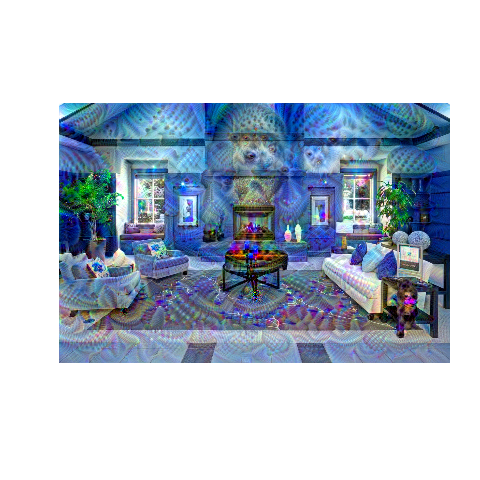Deep Dream InceptionV3 algorithm here is trained on ImageNet, then makes changes that increase confidence in the predicted category. Looped several times with the num_octave hyperparameter, it starts to look a good bit trippy and helps give some intuition what a neural network “sees” as prototypical examples of a predicted class. Apparently there is no “view of apartment” class as it keeps seeing ghastly animals. Perhaps it is no coincidence even before running InceptionV3 this clubhouse already looks like it could work in The Shining: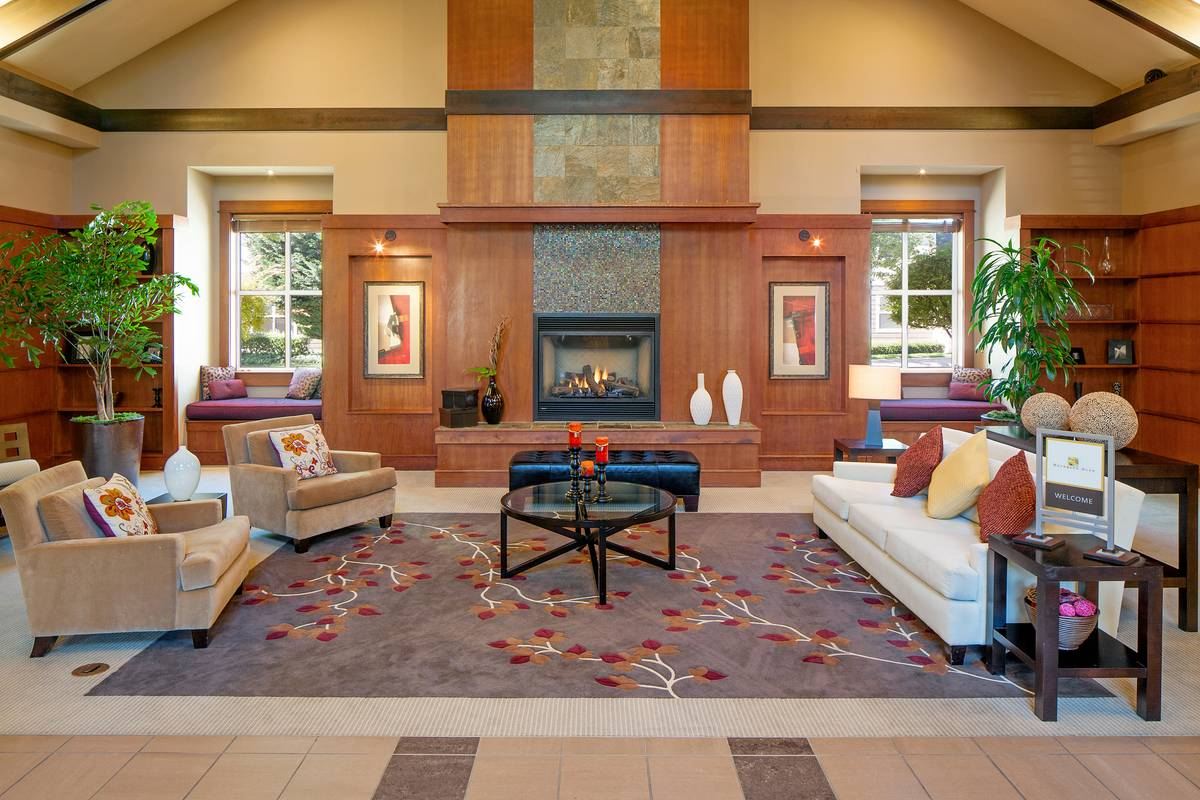### \$1850 / 1br - 697ft2 - BRAND NEW! Enjoy Luxury Uban [Urban?] Living at The Franklin Flats!

“NEW ON THE MARKET!

“The Franklin Flats is the newest addition to this desirable part of town! Built with the urban adventurer in mind, our small community offers luxury appeal with a neighborhood feel. Boasting a walkability score of 86 out of 100, you can’t beat the location! [unless an 87+?] Our close proximity to Mt. Tabor, food carts, [because you won’t have anything left over for restaurants] shopping and eateries gives you the classic Northwest experience you crave. Email or call to schedule a personal tour today!”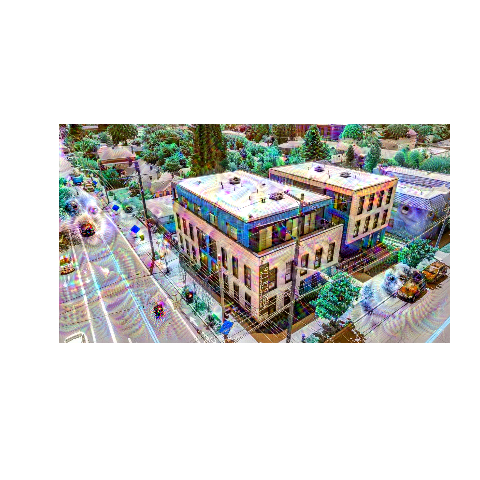Apparently the Attack on Titan seals make this a desirable part of town.

Perhaps those seals are why walkability doesn’t crack 90. If you survive the seals on a midday stroll, there are titan polar bears who can walk through walls: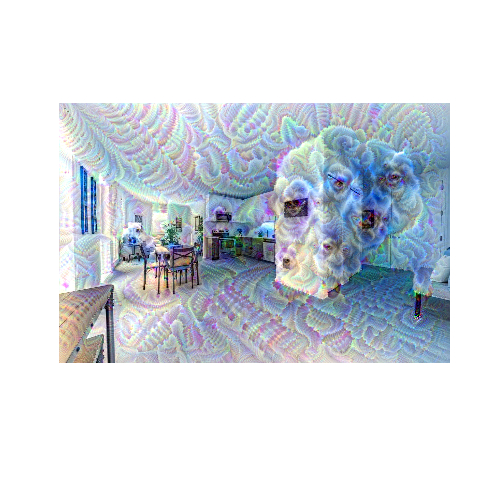### \$4250 / 2br - 1900ft2 - Condo on the Willamette

“Breathtaking views of the city and the Willamette River, located in the elegant Atwater. This condo has two bedrooms, living room, dining room, gourmet kitchen, gas fireplace, small office, two balconies, utility room and underground parking. Includes concierge desk, card-accessed security.”

Something tells me this view of the Willamette River would be complete if a cocker spaniel is staring at me…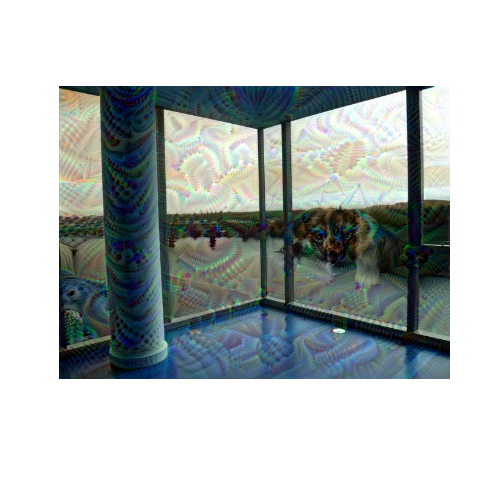But this view is what you really pay for: look at all the suckers in the two identical buildings who massively overpaid for – how the heck did those get up here?!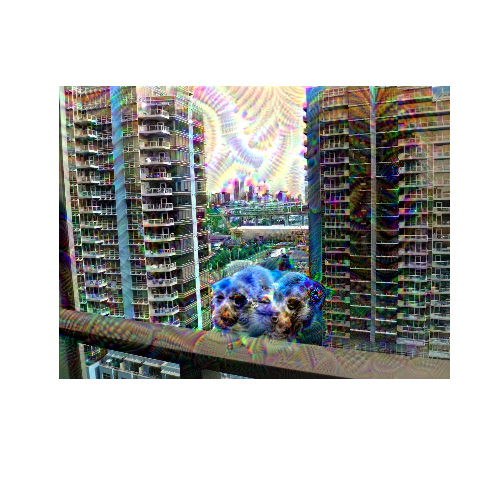### \$3900 / 2br - 1004ft2 - Portland’s most well-appointed two bedroom apartments now available

“Portland’s premier rental community is now pre-leasing. Find everything you desire in one place: The finest dining, distinguished boutiques, and most-beloved haunts. Experience the perfect merger of luxury and livability with our timeless brick exterior, stunning marble lobby, tiled bathrooms, tall ceilings, ample light, extensive storage, concierge services, and even a dog washing station.

“Proudly situated in Portland’s [S]Nob Hill/23rd Ave neighborhood, 21 Astor boasts a “97” walk score and a “96” bike score. [Beat that Franklin Flats!] Life is great in the epicenter of Portland’s charm. Pick up your locally-grown produce at City Market. Grab happy hour with the gang at Seratto. Sweat it out at Corepower Yoga.

“Our greater than 1:1 parking ratio assures a space for your car in our garage. Imagine never having to find parking on NW 23rd again.”

I work on NW 21st, that’s almost the cost of parking alone. People walk by on Oregon ‘pot tours’ and this may be how they see the building as well: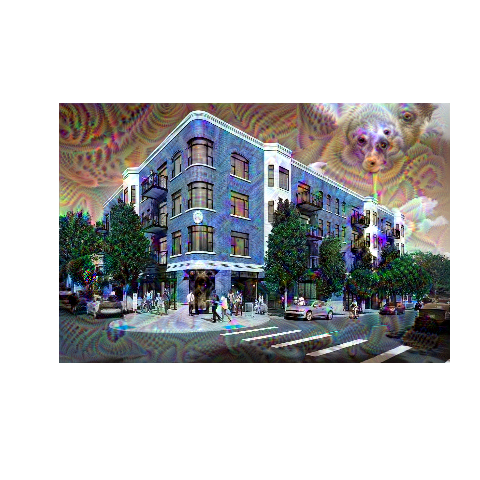Whoa! Is that a pig in the sky? Far out!

At least their new \$3.88/sqft/month kitchens aren’t yet haunted – oh, god! Are those giant psychedelic worms!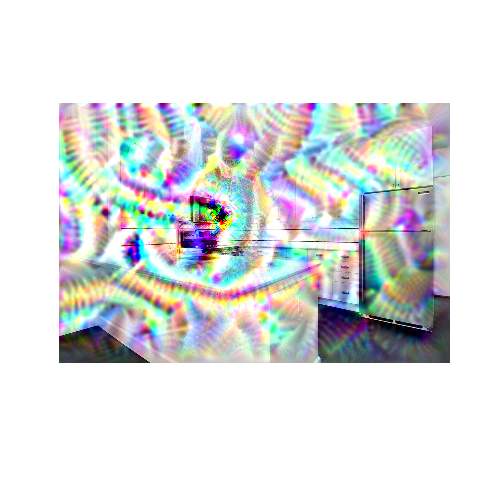## Code:

Started with JJ Allaire, François Chollet, RStudio, and Google’s keras code based on Google DeepDream. Added a little looping to try different parameters over these 7 images:

``````library(keras)
library(tensorflow)
library(purrr)

# Function Definitions ----------------------------------------------------

preprocess_image <- function(image_path){
image_to_array() %>%
array_reshape(dim = c(1, dim(.))) %>%
inception_v3_preprocess_input()
}

deprocess_image <- function(x){
x <- x[1,,,]

# Remove zero-center by mean pixel
x <- x/2.
x <- x + 0.5
x <- x * 255

# 'BGR'->'RGB'
x <- x[,,c(3,2,1)]

# Clip to interval 0, 255
x[x > 255] <- 255
x[x < 0] <- 0
x[] <- as.integer(x)/255
x
}

# Parameters --------------------------------------------------------

## list of images to process --
list_images <- list.files('images/deep dream apartments/orig/', full.names = TRUE)

## list of settings to try --
list_settings <- list(
settings = list(
features = list(
mixed2 = 0.2,
mixed3 = 0.5,
mixed4 = 2.,
mixed5 = 1.5),
hyperparams = list(
# Playing with these hyperparameters will also allow you to achieve new effects
step = 0.01,  # Gradient ascent step size
num_octave = 6,  # Number of scales at which to run gradient ascent
octave_scale = 1.4,  # Size ratio between scales
iterations = 20,  # Number of ascent steps per scale
max_loss = 10
)
),
settings = list(
features = list(
mixed2 = 0.5,
mixed3 = 0.2,
mixed4 = 1.1,
mixed5 = 1.5),
hyperparams = list(
step = 0.01,
num_octave = 9,
octave_scale = 1.1,
iterations = 20,
max_loss = 5
)
),
settings = list(
features = list(
mixed2 = 0.02,
mixed3 = 0.05,
mixed4 = 0.01,
mixed5 = 0.05),
hyperparams = list(
step = 0.01,
num_octave = 11,
octave_scale = 1.1,
iterations = 20,
max_loss = 20
)
),
settings = list(
features = list(
mixed2 = 0.2,
mixed3 = 0.5,
mixed4 = 2.,
mixed5 = 1.5),
hyperparams = list(
step = 0.01,
num_octave = 8,
octave_scale = 1.4,
iterations = 20,
max_loss = 10
)
),
settings = list(
features = list(
mixed2 = 0.2,
mixed3 = 0.1,
mixed4 = 0.4,
mixed5 = 0.3),
hyperparams = list(
step = 0.01,
num_octave = 8,
octave_scale = 1.4,
iterations = 20,
max_loss = 10
)
),
settings = list(
features = list(
mixed2 = 1.2,
mixed3 = 1.5,
mixed4 = 3.,
mixed5 = 2.5),
hyperparams = list(
step = 0.01,
num_octave = 8,
octave_scale = 1.4,
iterations = 20,
max_loss = 25
)
),
settings = list(
features = list(
mixed2 = 0.2,
mixed3 = 2.5,
mixed4 = 2.,
mixed5 = 3.5),
hyperparams = list(
step = 0.05,
num_octave = 6,
octave_scale = 1.4,
iterations = 20,
max_loss = 13
)
),
settings = list(
features = list(
mixed2 = 0.2,
mixed3 = 2.5,
mixed4 = 2.,
mixed5 = 3.5),
hyperparams = list(
step = 0.05,
num_octave = 8,
octave_scale = 1.4,
iterations = 20,
max_loss = 13
)
)
)

# Model Definition --------------------------------------------------------

k_set_learning_phase(0)

# Build the InceptionV3 network with our placeholder.
# The model will be loaded with pre-trained ImageNet weights.
model <- application_inception_v3(weights = "imagenet", include_top = FALSE)

# This will contain our generated image
dream <- model\$input

# Get the symbolic outputs of each "key" layer (we gave them unique names).
layer_dict <- model\$layers
names(layer_dict) <- map_chr(layer_dict ,~.x\$name)

## just loop on images - bottleneck is training anyway --
for(image_path in list_images){

image <- preprocess_image(image_path)

#res <- predict(model,image)
#reduce(dim(res)[-1], .f = `*`) %>%
#  as.numeric() %>%
#array_reshape(res, c(2, 51200)) %>%
#  imagenet_decode_predictions()
## get model prediction:
#x <- image_to_array(image)
#x <- array_reshape(image, c(1, dim(image)))
#x <- imagenet_preprocess_input(image)
#preds = predict(model, x)
#imagenet_decode_predictions(preds, top = 3)
#predict_classes(model, image)
# need to try again later

setting_counter <- 0

## then nested loop on settings --
for(settings in list_settings){
setting_counter <- setting_counter + 1
print(paste0('starting ', image_path, ', setting # ',  setting_counter))

image <- preprocess_image(image_path)

# Define the loss
loss <- k_variable(0.0)
for(layer_name in names(settings\$features)){

# Add the L2 norm of the features of a layer to the loss
coeff <- settings\$features[[layer_name]]
x <- layer_dict[[layer_name]]\$output
scaling <- k_prod(k_cast(k_shape(x), 'float32'))

# Avoid border artifacts by only involving non-border pixels in the loss
loss <- loss + coeff*k_sum(k_square(x)) / scaling
}

# Compute the gradients of the dream wrt the loss

# Set up function to retrieve the value
# of the loss and gradients given an input image.
# this function will crash the R session if too many octaves on too small an image

list(
loss_value = outs[],
)
}

gradient_ascent <- function(x, iterations, step, max_loss = NULL) {
for (i in 1:iterations) {
if(is.na(out\$loss_value)){
print(paste0('NA loss_value on setting # ', setting_counter))
break
} else if (!is.null(max_loss) & out\$loss_value > max_loss) {
break
}
print(paste("Loss value at", i, ':', out\$loss_value))
x <- x + step * out\$grad_values
}
x
}

original_shape <- dim(image)[-c(1, 4)]
successive_shapes <- list(original_shape)

for (i in 1:settings\$hyperparams\$num_octave) {
successive_shapes[[i+1]] <- as.integer(original_shape/settings\$hyperparams\$octave_scale^i)
}
successive_shapes <- rev(successive_shapes)

original_image <- image
shrunk_original_img <- image_array_resize(
image, successive_shapes[], successive_shapes[]
)

shpnum <- 0 # for debugging
for (shp in successive_shapes) {
shpnum <- shpnum + 1 # for debugging

image <- image_array_resize(image, shp, shp)
print(paste0('running octave ', shpnum))# for debugging
settings\$hyperparams\$max_loss)
print(paste0('finished octave ', shpnum))# for debugging
upscaled_shrunk_original_img <- image_array_resize(shrunk_original_img, shp, shp)
same_size_original <- image_array_resize(original_image, shp, shp)
lost_detail <- same_size_original - upscaled_shrunk_original_img

image <- image + lost_detail
shrunk_original_img <- image_array_resize(original_image, shp, shp)
}

image_path %>%
gsub('/orig/', '/dream/', .) %>%
gsub('.jpg', paste0('_dream', setting_counter, '.png'), .) %>%
png(filename = .)
plot(as.raster(deprocess_image(image)))
dev.off()
print(paste0('finished ', image_path, ', setting # ',  setting_counter))
}
}
``````
Written on July 6, 2018